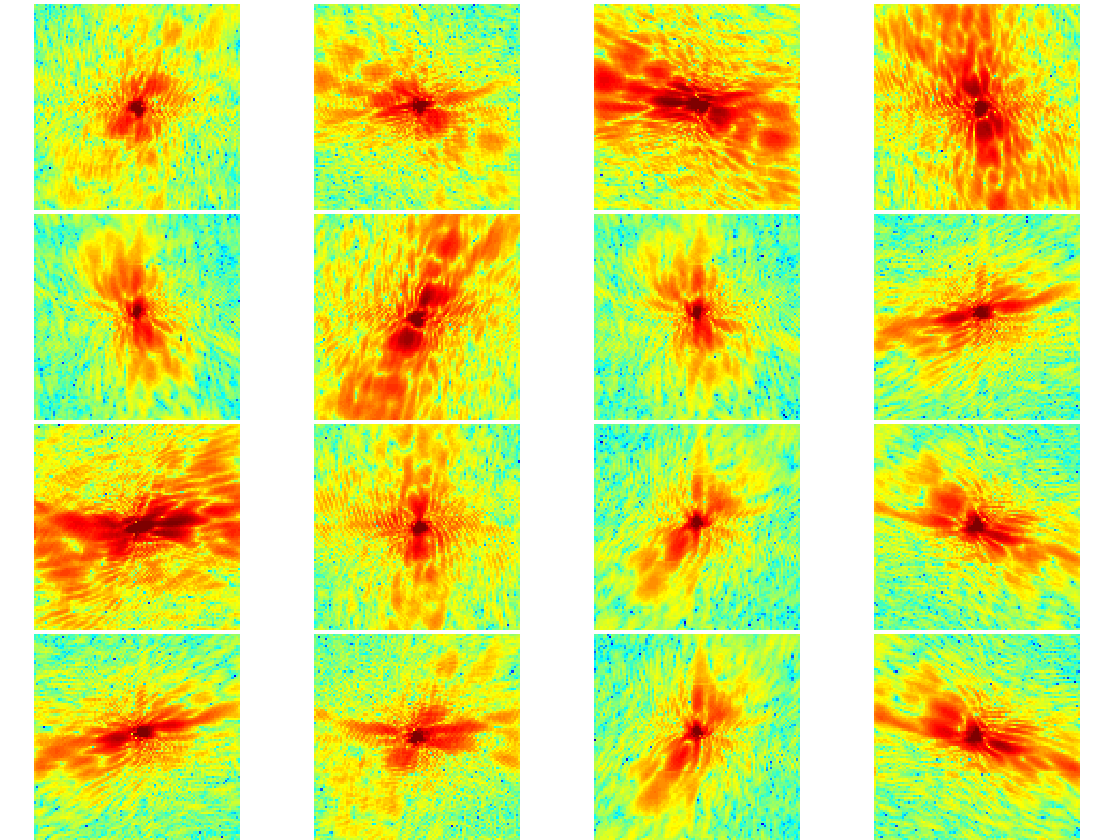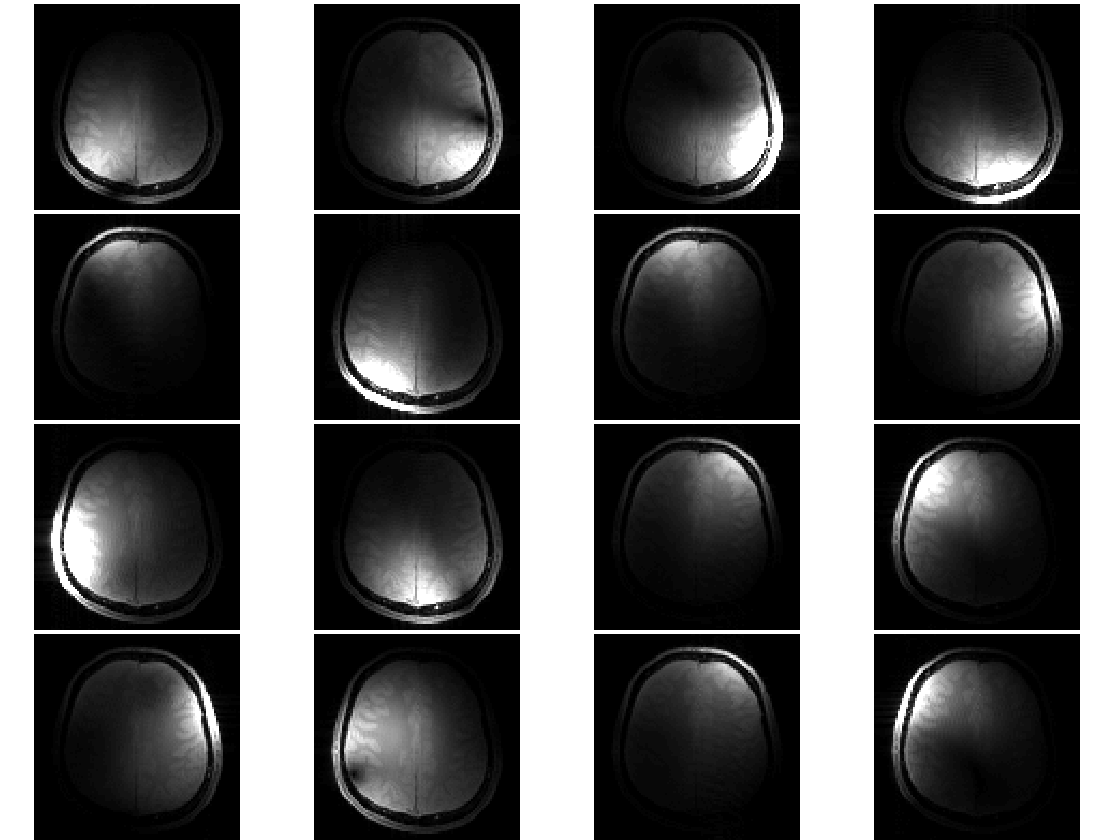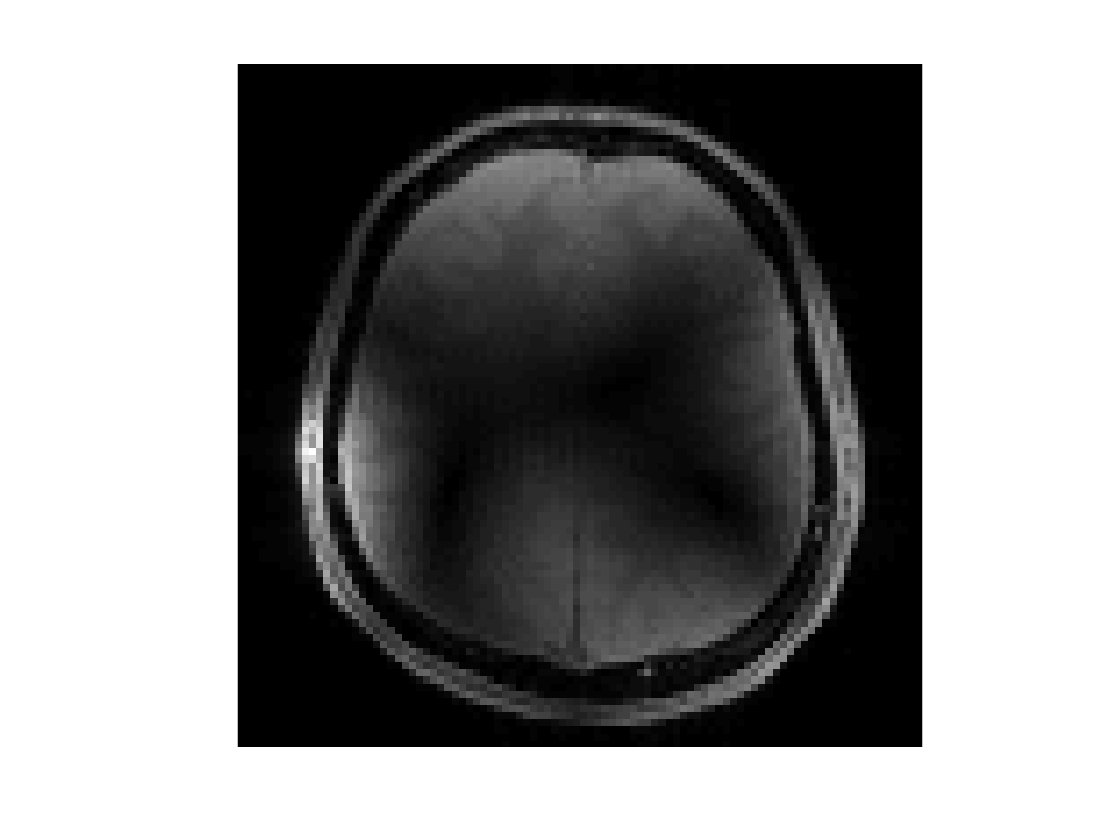# SENSE Parallel Imaging

Mark Chiew (mark.chiew@ndcn.ox.ac.uk)
This MATLAB tutorial gives an introduction to SENSE parallel imaging in MRI. It walks through the estimation of coil sensitivities, combining images from multiple coils, and reconstruction of under-sampled data using the SENSE algorithm.
The data required for this can be downloaded from:
users.fmrib.ox.ac.uk/~mchiew/docs/raw_data.mat

## Explore and view sensitivities

There is a single raw 4D array containing the data we'll be working with, named raw
[Nx,Ny,Nz,Nc] = size(raw)
Nx = 96
Ny = 96
Nz = 1
Nc = 16
The dimensions of the dataset are (Nx, Ny, Nz, Nc) where (Nx, Ny, Nz) correspond to the spatial image matrix size, and Nc corresponds to the number of difference receiver coils ( or coil channels).
In this case, we're working with a 96x96 2D image (Nz=1), that has 16 different coils.
The raw data are provided exactly as measured - which means this is complex k-space data. We get one k-space dataset per coil, which we can view the magnitude of:
show_grid(log(abs(raw)), [-2 8], jet)How do the k-space data for each coil look similar/different?
The coil in the botton right (coil #16), for example, and the one right above it (coil #15) look very similar in k-space magnitude. Do we expect them to look the same in image space?
To look at the images, we need to transfom the k-space data into image space, via the inverse discrete Fourier Transform (DFT). The FFT (and its inverse iFFT) are the most efficient ways to compute DFTs. We have defined some helper functions to do this properly for us - they just make sure that our imaging conventions match up with MATLAB's FFT conventions:
img = ifft2c(raw);
show_grid(abs(img),[0 16], gray);• How do the images look different?
• What do you think this tells you about the physical coil array?
• Do you notice anything about the k-space magnitude data that tells you about the coil images?

## Combine images

Before we talk more about coil sensitivities, lets consider the simple problem of combining our multiple coil images into a single representative brain image.
That means we want to define a transform that takes our [Nx, Ny, 1, Nc] data and maps it to a single [Nx, Ny, 1, 1] 2D image. For example, we could just take the mean:
img_combined = mean(img,4);
show_img(abs(img_combined),[]);This image is clearly not an accurate representation of what we expect this brain slice to look like. What we would like a coil combination to produce is an image that does not actually have any coil-specific weighting or influence - we would to see what the brain looks like, not what the coils look like.
• What are the problems here, and why do they occur?
• Can you come up with a better coil-combination transform?
Hint: Try transforms of the form:% define and view your own coil-combine transforms
% [Nx, Ny, 1, Nc] -> [Nx, Ny]
% Try linear and non-linear operations!
% e.g.:
img_combined = sqrt(sum(abs(img).^2,4));
show_img(abs(img_combined),[]);